# Monoatomic linear lattice

A monoatomic linear lattice refers to a one-dimensional lattice structure composed of a single type of atom. In this lattice, the atoms are arranged in a linear, one-dimensional array.

Each atom in the lattice occupies a specific position along the line, and the arrangement repeats periodically. The spacing between adjacent atoms in the lattice is known as the lattice constant or lattice spacing.

The lattice constant in a monoatomic linear lattice can be represented by the symbol "a." It defines the distance between neighbouring atoms and plays a crucial role in determining the properties of the lattice, such as the spacing of diffraction peaks in X-ray or neutron diffraction experiments.

A monoatomic linear lattice can be described using the Bravais lattice concept, which characterizes the translational symmetry of the lattice structure. In the case of a linear lattice, the Bravais lattice is typically represented by a one-dimensional lattice vector.

Monoatomic linear lattices are simplified models used to study the behaviour of specific materials or to understand fundamental concepts in solid-state physics. They serve as building blocks for more complex crystal structures and can provide insights into the properties and behaviour of materials in certain directions. Let us consider one dimensional linear chain of monoatomic crystal having mass of each atom 'm' and lattice constant 'a'. Suppose that these atoms are connected by an ideal spring having spring constant 'c'. Let (n-3)th, (n-2)th......(n+3)th are positions of atoms before vibration and Un+3, Un+2........be displacement of these atoms from equilibrium position after vibration.

A we are considering the elastic response of the crystal, force experienced by the nth atom is proportional to displacement. We also consider the nearest interatomic interaction.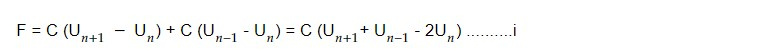From newton' s second law of motion,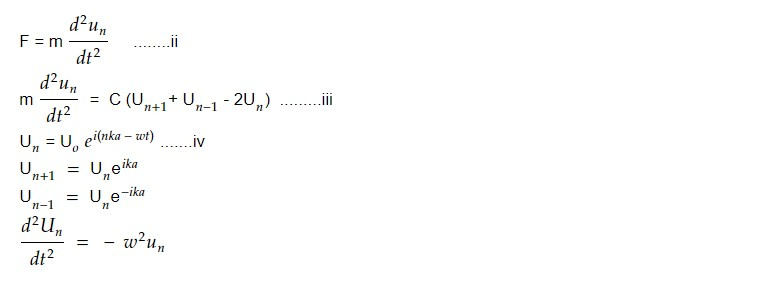So equation iii becomes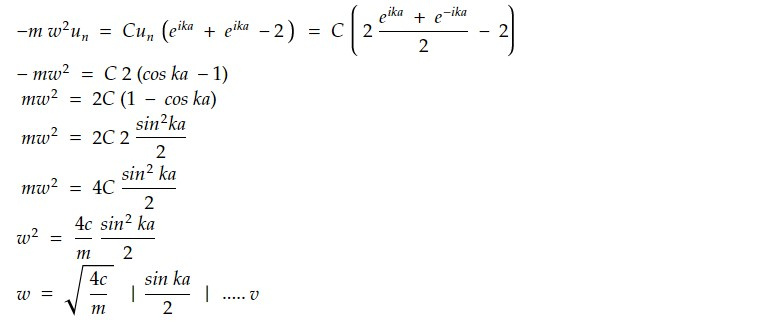At low frequency range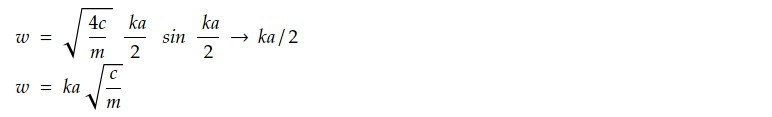Particle velocity Vp = w/ k = a c/m

Group velocity Vg = dw/dk = a c/m

The relation v= w/k is same as that for a homogeneous continuous line thus discrete nature of structure is not sensitive to long wavelength. The medium is no more dispersive.

2. At high frequency range: At high frequency, the phase velocity.

Vp = w/kAs a result, both velocities depend on frequency. This is comparable to an optical example in which light passes through a medium and the phenomenon of light dispersion is caused by the refractive index, which is a function of frequency. By analogy, it can be claimed that the current medium is dispersive.

B. At frequency w = 4c/m

From the relation, w = 4c/m sin ka/2

Frequency becomes maximum at sin ka/2 = 1 = sin π/2

k = π/a

2π/ λ = π/a

or, λ = 2a

From Bragg's law, nλ =2 dsin θ if n=1, θ = 90°.

Vp = w/k = Wmass / k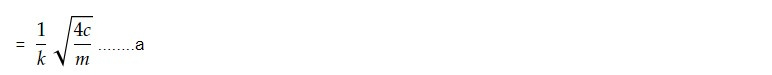We know linear density,

ρ = m/a

=> m = ρ / a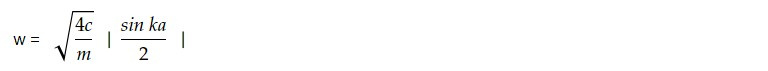Put c = E/a

where E is longitudinal stiffness constant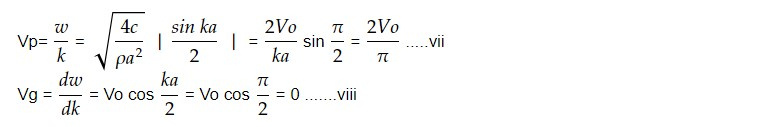From vii and viii, we find that the group velocity is zero and phase velocity is 2Vo/ π. The group velocity represents the velocity of energy transmission or signal.

This note is a part of the Physics Repository.